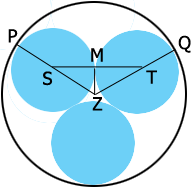SEARCH HOMEMath Central Quandaries & QueriesQuestion from renu, a parent: inside of a circle K of radius length measure R,three circular discs A,Band C each of radius r are placed so that each touches the other two and K . express R in terms of r. in the space between K, A and B , another circular disc D is placed which just touches K, A and B. if the radius is s, show that (6+root3)s=(2+root3)rHi REnu,

I can help you with the first task, expressing R in terms of r.

In my diagram $Z$ is the centre of the circle $K$ and $S$ and $T$ are the centres of circles $A$ and $B.$ $M$ is the midpoint of the line segment $ST.$The length $|TQ| = r$ and the length $|ZQ| = R$ so if you can express the length $|ZT|$ in terms of $r$ you have what you need.

What is the measure of the angle $SZT?$

What is the measure of the angle $MZT?$

What is the measure of the angle $TMZ?$

$|MT| = r.$ What is the length $ZT?$

PennyMath Central is supported by the University of Regina and The Pacific Institute for the Mathematical Sciences.